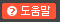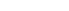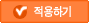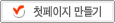# 태터데스크 관리자# 태터데스크 메시지

저장하였습니다.

## 투명 필름 문제(Scheme)

Tech 2008. 8. 1. 08:44
코딩도장에서 풀고 있는 투명 필름 문제. UVA 에서 뽑아온 문제인데 ... 그쪽 스타일은 입출력이 귀찮아서 열심히 하기는 싫다. 그냥 내맘대로 문제를 줄여서(-_-a) scheme 으로 뚝딱. 아직 공부가 부족한가보다. ... 더 잘 할 수 있을텐데. 위키보다는 여기가 어울릴 것 같아서(문서가 아니라 코드!) 붙임. SICP 스타일로,

Representation 정하기
Selector 등 Abstraction layer 만들기
Logic 구현

의 순서를 밟았다. 실제로 Abstraction layer를 만들어둔게 디버깅 할때 꽤나 큰 도움이 되었다. <시작, 끝> 에서 시작이 끝보다 작은 필름의 경우 make-film을 고치는 것 만으로 해결된 것.

-----
`; My film system!(define (make-film s e t)  (if (> s e)      (list e s t)      (list s e t)))(define (start-point film) (list 's (car film) (transparency film)))(define (end-point film) (list 'e (cadr film) (transparency film)))(define (start-point? point) (eq? 's (car point)))(define (end-point? point) (eq? 'e (car point)))(define (point-pos point) (cadr point))(define (point-transparency point) (caddr point))(define (transparency film) (caddr film)); Test cases!(define test-case-1 (list (make-film 2.0 9.0 0.9)                          (make-film 4.0 13.5 0.7)                          (make-film 7.0 17.0 0.8)))(define test-case-2 (list (make-film 6.0 24.0 0.9)                          (make-film 5.5 22.0 0.7)                          (make-film 9.0 18.0 0.8)                          (make-film 16.0 16.0 0.9)                          (make-film 8.5 15.0 0.7)                          (make-film 4.0 11.0 0.8)                          (make-film 2.2 5.0 0.9)                          (make-film 90.5 1.0 0.7)))(define test-case-3 (list (make-film 1.0 2.0 0.5)                          (make-film 1.0 3.0 0.5)                          (make-film 2.0 3.0 0.5))); Main solver(define (solve films)  ; Input manager  (define (adjoin-point-list p l)    (cond ((null? l) (list p))          ((< (point-pos p) (point-pos (car l))) (cons p l))          ((or (> (point-pos p) (point-pos (car l))) (= (point-pos p) (point-pos (car l))))           (cons (car l)                 (adjoin-point-list p (cdr l))))          (else           (error "Error!"))))  (define (make-point-list l)    (cond ((null? l) '())          ((= (point-pos (start-point (car l))) (point-pos (end-point (car l))))           (make-point-list (cdr l))) ; for a vertical film          (else           (adjoin-point-list (start-point (car l))                              (adjoin-point-list (end-point (car l))                                                 (make-point-list (cdr l)))))))  (define expanded-input (make-point-list films)) ; This is the very input for main solver function  ; Utility functions for main solver  (define (add-film cur-transparency added-transparency)    (* cur-transparency added-transparency))  (define (sub-film cur-transparency added-transparency)    (/ cur-transparency added-transparency))  ; Main solver  (define (iter output before cur-trs remaining_input)    (let ((next-point (if (null? remaining_input)                          '() ; It's not gonna be used                          (car remaining_input))))      (if (null? remaining_input)          output          (iter (append output (list (list before (point-pos next-point) cur-trs)))                (point-pos next-point)                (if (start-point? next-point)                    (add-film cur-trs (point-transparency next-point))                    (sub-film cur-trs (point-transparency next-point)))                (cdr remaining_input)))))  ; Direct output from main solver. It has some duplicates and useless info  (define raw-output (iter '() 0 1 expanded-input))  ; Filter out some meaningless output element  (define output-filter    (lambda (sequence)      (filter (lambda (x)                (< (car x) (cadr x)))              sequence)))  ; Combine(Reduce) some outputs for compact result  (define (combinable? x y)    (and (= (cadr x) (car y))         (= (caddr x) (caddr y))))  (define (combine x y)    (list (car x) (cadr y) (caddr x)))  (define output-reducer    (lambda (sequence)      (cond ((null? (cdr sequence)) sequence)            ((combinable? (car sequence) (cadr sequence))             (cons              (combine (car sequence) (cadr sequence))              (cddr sequence)))            (else (cons                   (car sequence)                   (output-reducer (cdr sequence)))))))  ; So, this is my final output!  (output-reducer (output-filter raw-output)))-----ordered-list 에 대한 일반적 구현을 만들어서 분리하면 코드가 확 줄어들 것 같다.`

#### 'Tech' 카테고리의 다른 글

 하위 디렉토리에 있는 모든 .svn 을 날리는 법  (5) 2008.09.11 2008.08.09 2008.08.01 2008.07.22 2008.07.18 2008.06.11
1.comkid 2008.08.01 09:39

scheme으로도 코드가 상당히 길게 나온다고 생각했는데 테스트 케이스가 포함되서 그런거군요..
왠지 손으로 써보지 않고는 해석이 안 될 것 같은.. ~_~

2.슈레인 2008.08.01 11:03

uva는 정말 입력이 즐 -_-

3.deisys 2008.08.01 15:00

comkid // 제가 능력이 딸려서 코드가 긴겁니당... ㅠㅠ
슈레인 // 그러게... 탑코더가 좋아~

4.ugg boots 2013.07.14 01:41

지금은 반짝반짝 빛이 나겠지,, 하지만 시간이 흐르면 그빛은 사라저버릴거야,지금 우리처럼